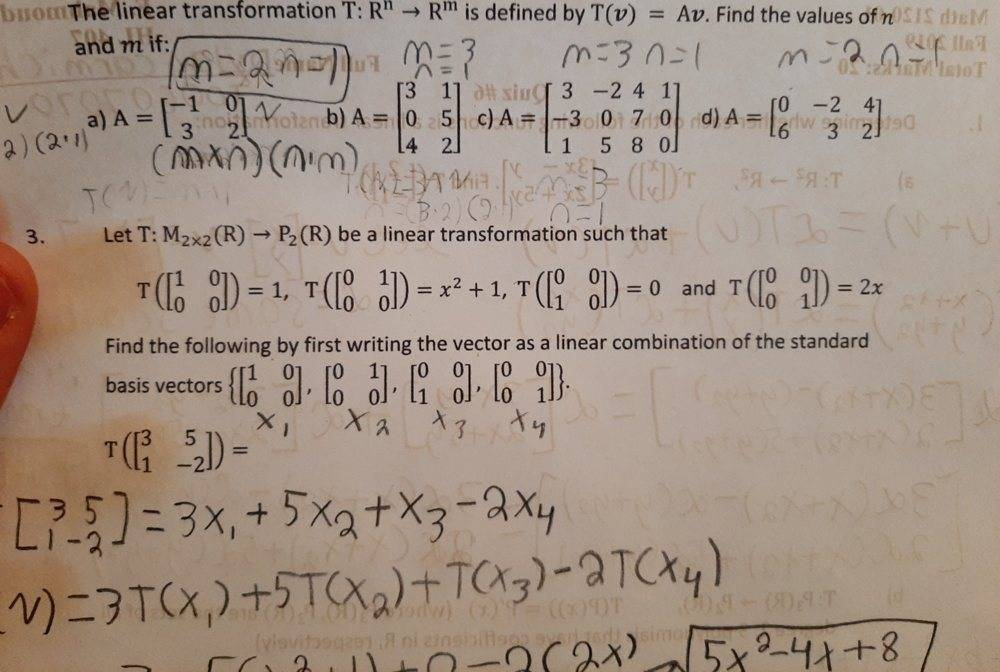# Find n and m values of a linear Transformation if given a matrix A

Homework Statement:
The linear transformation T: R^n---> R^m is defined by T(v)=Av. Find the values of n and m if A is the following matrix. How do i find n and m values?
Relevant Equations:
T(v)=AvMark44
Mentor
Homework Statement: The linear transformation T: R^n---> R^m is defined by T(v)=Av. Find the values of n and m if A is the following matrix. How do i find n and m values?
Homework Equations: T(v)=Av

View attachment 251993
For a matrix product Ax, where A is an m X n matrix (m rows and n columns) and x is an n X 1 matrix (a column vector), the product will be an m X 1 vector. In the problem, it's given that ##T:\mathbb R^n \to \mathbb R^m##, so x has to belong to which of these spaces, and T(x) has to belong to which of these spaces?
BTW, your doodling on the paper in the image doesn't come anywhere close to the answers to problem 2.

For a matrix product Ax, where A is an m X n matrix (m rows and n columns) and x is an n X 1 matrix (a column vector), the product will be an m X 1 vector. In the problem, it's given that ##T:\mathbb R^n \to \mathbb R^m##, so x has to belong to which of these spaces, and T(x) has to belong to which of these spaces?
BTW, your doodling on the paper in the image doesn't come anywhere close to the answers to problem 2.
x Has to belong to Rn and T(x) must belong to Rm

lekh2003
Gold Member
Why don't you try some random matrices with different sizes and see what happens? Experimenting, and then learning from what doesn't work can help you derive how to solve problems of this type.

Mark44
Mentor
x Has to belong to Rn and T(x) must belong to Rm
Right.
In problem 2b of the image you posted, it has ##A = \begin{bmatrix} 3 & 1 \\ 0 & 5 \\ 4 & 2\end{bmatrix}##, and A is a 3 X 2 matrix (m = 3, n = 2). Above it you wrote m = 3 and n = 1, which isn't correct. If x is a column matrix to the right of A, it needs to be <how big?> X 1? The number you get here is the dimension of the domain space.

And the result vector needs to be <how big?> X 1? The number here will be the dimension of the range space.

Right.
In problem 2b of the image you posted, it has ##A = \begin{bmatrix} 3 & 1 \\ 0 & 5 \\ 4 & 2\end{bmatrix}##, and A is a 3 X 2 matrix (m = 3, n = 2). Above it you wrote m = 3 and n = 1, which isn't correct. If x is a column matrix to the right of A, it needs to be <how big?> X 1? The number you get here is the dimension of the domain space.

And the result vector needs to be <how big?> X 1? The number here will be the dimension of the range space.
And x must be a vector of nx1 which will result in a 3x1 matrix in b so its going from R^2 from 3 to R^3